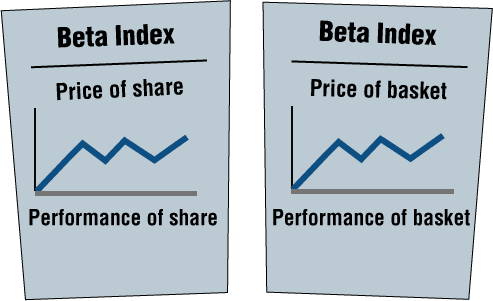# Describing Behavior of Shares Using the β (beta) Index

A stock’s β reflects changes in share price during a previous period (for example, the past year), as compared with the behavior of the price of the basket during that same period.

A stock’s β is calculated using a formula that considers the performance of the share and the performance of the basket throughout the entire period.

We will not present the formula here, but we will examine the meaning of the results with respect to each of the four groups described above. We will also assume that any given shareís future behavior will be identical to its behavior during the previous period.

Examples

Group 1

When we obtain β = 2 for some share in Group 1, it means that:

1. On days when the price of the basket rises, we expect the price of the share to rise twice as much. If the price of the basket rises 1.1% on a given day, we expect the price of the share to rise by 2.2% (twice as much).

2. On days when the price of the basket falls, we expect the price of the share to fall twice as much. If the price of the basket falls by 0.6% on a given day, we expect the price of the share to fall twice as much, i.e. by 1.2%.

β is greater than 1 for all of the shares in Group 1.

Illustrator 5.1 summarizes the explanation for this.Group 2

β is less than 1 for all of the shares in Group 2. This means that when the price of the basket rises by some percentage, the price of the share also rises, but to a lesser extent.

For example, if the β of some share is 0.7, this means that if the price of the basket rises by 1% on a given day, then we can expect the price of the share to rise by 0.7% (1% X 0.7).

Group 3

β is negative for all of the shares in Group 3.

For example, β can be -1.5 or -0.7.

The meaning of the negative number is that the share behaves in the opposite manner as compared with the price of the basket.

The further the share moves in the opposite direction from the price of the basket, the larger the negative number that will result (-3 reflects more opposite behavior than -2). When β = -2, it means that when the price of the basket rises by a given percentage (for example, 1%), the share can be expected to fall by twice as much as the price of the basket (-2%).

On the other hand, if the price of the basket fell by a given percentage (for example, 0.3%), the price of the share can be expected to rise by twice as much as the basket fell (+0.6%).

Group 4

We will leave the specific meaning of β as it relates to shares in this group to those with previous knowledge of the subject.